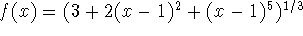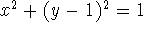Next: About this document Up: MA1022 Lab 3 - Previous: Background

## Exercises

1. This exercise is intended to familiarize you with the procedures revolve, RevInt, LeftDisk and LeftInt as an approach to approximating the volume of a solid of revolution using the disk method. Apply the four commands as listed above to the function f defined byon the interval [0,2] with n=10. Your answer should include the two numerical values and references to the two 3-dimensional plots.
2. A torus - a solid shaped like a doughnut - is generated by revolving a circle about an axis that does not intersect the circle. By rotating the circleabout the line y = 3, print a plot of the torus which depicts its doughnut-like image and compute its volume. Your answer should include the numerical value for the volume and a reference to the 3-dimensional plot.
3. How would you generalize the formula given in the background to find the volume of a solid generated by revolving the area between a segment of the graph of a function and an arbitrary line parallel to the x-axis?
4. Design a drinking glass by revolving a suitable function about the x-axis. In your report, give the function, a three dimensional plot of your glass and calculate the volume of the liquid filled portion. You will be graded on the utility and elegance of your design.

Roxanne Tisch
Thu Apr 24 09:03:12 EDT 1997